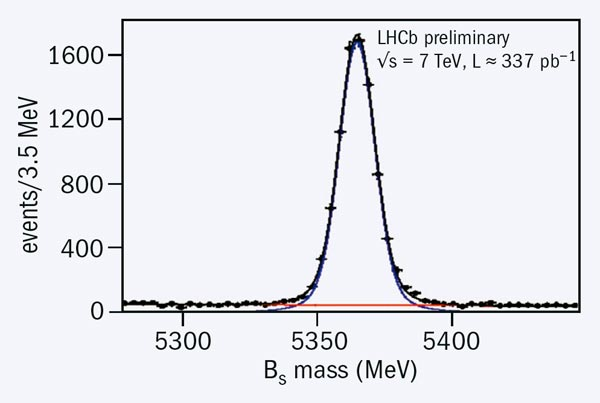# LHCb pins down the Bs mixing phase

23 September 2011Fig. 1. Preliminary results for the width difference ΔΓs vs the mixing phase φs from DØ (Burdin 2011), CDF (CDF 2010) and LHCb (LHCb 2011a). The contours have been redrawn and overlaid for comparison here, the original plots can be found in the references.

The LHCb collaboration’s presentation at Lepton Photon 2011 included one of the most eagerly awaited measurements in flavour physics: the CP violation phase in BsB mixing. This is the counterpart of sin 2β in the B0 system, which was measured by the B-factory experiments BaBar and BELLE using the channel B0→ J/Ψ Ks. They provided the first measurement of CP violation in B0 mixing, which is both large and now well measured, with sin 2β = 0.69 ± 0.02. In contrast, the Standard Model prediction for φs, the corresponding phase for the Bs meson, is extremely small and precise: φs = 0.036 ± 0.002 rad (Charles et al. 2005). It is therefore an interesting place to search for new physics beyond the Standard Model, which may enhance the value. Time-dependent analyses of Bs mesons were not accessible at the B-factories, so this remained a key measurement for hadronic machines, first at the Tevatron and now at the LHC.

The golden mode for this study is Bs → J/Ψ φs where the J/Ψ decays to μ+μ and the φ decays to K+K . The measurement is very challenging: the final state is not a pure CP eigenstate, so an angular analysis has to be made to separate the CP-even and CP-odd components. In addition, the fast BsBs oscillation necessitates precise vertex reconstruction, and tagging of the production state (whether it was a Bs or Bs ) is also important. The result for φs is correlated to another quantity in the fit, ΔΓs, the difference in width of the two Bs mass eigenstates. (It is the mass difference of these two states that determines the oscillation frequency.) ΔΓs can be positive or negative, but in the Standard Model is predicted to be 0.087 ± 0.021 ps–1 (Lenz and Nierste 2011). The uncertainties on φs and ΔΓs are correlated, and furthermore the fit turns out to be insensitive to the replacement φs → π – φs when ΔΓs → – ΔΓs so there are two ambiguous solutions. As a result, the measurements are usually plotted as contours in the φs vs ΔΓs plane.

The CDF and DØ experiments at the Tevatron made the first measurements. Their early results agreed with each other and appeared, when combined, to indicate a large value for φs, about 3σ away from the Standard Model expectation. More recent updates have moved their preferred values somewhat closer to the Standard Model, but a hint of a possible discrepancy remained, as shown by the red and green contours in figure 1 (Burdin and DØ 2011, CDF 2010).Fig. 2. Signal for Bs → J/Ψ φ (LHCb 2011a). The line indicates the fitted background level.

LHCb has now accumulated the largest sample of Bs → J/Ψ φ decays in the world, over 8000 signal candidates with very high purity (figure 2). The resulting constraint is shown as the blue contour in figure 1 (LHCb 2011a). It is much more precise than the preceding measurements, with one of the two solutions being in good agreement with the Standard Model expectation – the hint of a discrepancy is not confirmed. This result also gives the first significant direct measurement of ΔΓs, 0.123 ± 0.029 ± 0.008 ps–1, where the first uncertainty is statistical and the second systematic.

Another related analysis presented by LHCb uses a different decay mode, Bs → J/Ψ f0, which should measure the same phase. Although the statistics are lower, the final state is CP-odd in this case, so the analysis is simpler (LHCb 2011b). It gives a consistent result to B0s → J/Ψ φ, and the preliminary combined result from LHCb is φs = 0.03 ± 0.16 ± 0.07 rad (LHCb 2011c). This result is statistically limited, but as data continue to pour in from the LHC there are good prospects for substantial further improvement. So, although LHCb has now ruled out a gross effect from new physics, the experiment should be able to measure the true value even if it is as small as predicted in the Standard Model – and test any subtle effects from new physics.